# 基础知识

## 一. 概率论基础

1. 条件概率公式：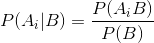1. 全概率公式：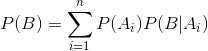1. 由条件概率公式和全概率公式可以导出贝叶斯公式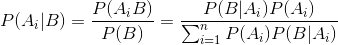## 二. 文本分类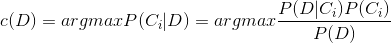c(D) = argmax P(D|C_i) * P(C_i)


## 三. 朴素贝叶斯分类模型

P(D|C, D_j) = P(D_i|C)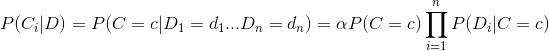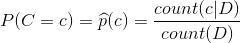count(c|D)：类别c中的训练文本数

count(D)：总训练文本数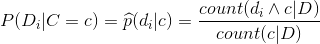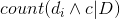单词di在类别c中出现的次数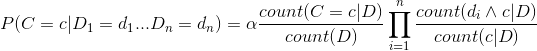# 朴素贝叶斯理论：

## 1. 分类规则: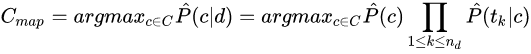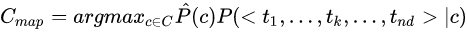## 2.条件独立性假设：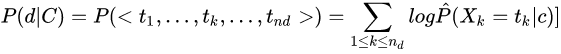## 3. 位置独立性假设: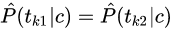1. 互信息

2. x^2 统计量

3. 词频统计

# 朴素贝叶斯的实现方式比较：

## 1、贝努利模型（Multivariate Bernoulli Model)：## 2、多项式模型：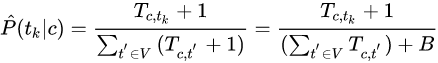# 朴素贝叶斯分类器（Naive Bayes Classifier ）：

## 1. 朴素贝叶斯是一个概率分类器n_d 是文档的长度（词条个数）

P(t_k | c) 是词项 t_k 出现在类别 c 中文档的频率，即类别 c 文档的一元语言模型

P(t_k | c) 度量的是当 c 是正确类别时 t_k 的贡献

p(c)是类别 c 的先验概率

## 2. 对数计算：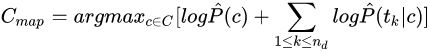ps: 这个转换非常重要，而且性能较好。分词时其实也有类似的操作。

## 3. 最大似然估计

(1) 先验概率 P(c) = Nc/N

Nc: 类 c 中的文档数目； N ：所有文档的总数

（2）条件概率：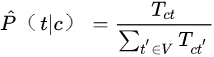T_ct 是训练集中类别 c 中的词条 t 的个数（多次出现要计算多次）

## 4. MLE估计的零概率问题：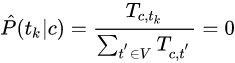## 5. laplace 平滑B是不同词语个数（这种情况下 |V| = B ）,利用加1平滑从训练集中估计参数

# 文本分类器评估(Classifier Evaluation)

## 2. 评估指标：

F_1 值是在正确率和召回率之间达到某种平衡

F_1 = 2PR / (P+R)

F_1 也就是P和R的调和平均值:

1/F_1 = 1/2 * (1/P + 1/R)

（1）数据预处理

（2）特征数据

（3）直接根据贝叶斯计算

# 参考代码

## 核心分类代码

import java.io.File;
import java.io.IOException;
import java.util.Vector;

public class TrainDataManager {
private static final String dirName = "trainingData/Sample"; // 训练集所在目录
CountDirectory countDir = new CountDirectory();
private int zoomFactor = 5; // 放大倍数

/**
* 计算先验概率 p(ci)=某个类别文章数/训练文本总数
*
* @param className 类别名称
*
* @return 类别的先验概率
*/
public double priorProbability(String className) {
double probability = 0.0;
probability = (double) countDir.countClass(className) / countDir.countSum();
return probability;
}

public void execute(String article) throws IOException {
// 进行分词
Vector<String> strs = ChineseSpliter.splitWords(article);

File dir = new File(dirName);
File[] files = dir.listFiles(); // 目录下的所有文件
String className;
double countc;
double product = 1;
Vector<Double> probability = new Vector<Double>();
double temp;
// 计算文本属于每个类别的概率
for (File f : files) {
className = f.getName();
countc = countDir.countClass(className);
// 计算文本中某个词属于特定类别中的概率
for (String word : strs) {
temp = (countDir.countWordInClass(word, className) + 1)
/ countc * zoomFactor;// 避免所得结果过小，对结果进行放大
product *= temp;
}
product = 1;
}

double max = 0;
int maxId = 0;
for (int i = 0; i < files.length; i++) {
if (max < probability.get(i)) {
max = probability.get(i);
maxId = i;
}
}
System.out.println("文章所属分类为：" + files[maxId].getName());
}
}


## 用于计算训练集中的各种频次

import java.io.BufferedReader;
import java.io.File;
import java.io.FileInputStream;
import java.io.IOException;

/**
* 计算各种频次
*
*
*/
public class CountDirectory {
private static final String dirName = "trainingData/Sample"; // 训练集所在目录

public int countSum() {
File dir = new File(dirName);
File[] files = dir.listFiles(); // 目录下的所有文件
String subName = ""; // 子目录的路径名称
int sum = 0; // 训练集中所有类别的总文本数

// 计算所有文件的总数
for (int i = 0; i < files.length; i++) {
subName = files[i].getName();
sum += countClass(subName);
}
return sum;
}

/**
* 用于计算某个类别下的文章总数
*
* @param className
*            类别名称
* @return 给定类别目录下的文件总数
*/
public int countClass(String className) {
String classPath = dirName + "/" + className;
File subDir = new File(classPath);// 子目录
File[] subFiles = subDir.listFiles(); // 子目录下的所有文件
return subFiles.length;
}

/**
* 计算某个类别中包含给定词的文章数目
*
* @param word
*            给定的词
* @param className
*            类别名称
* @return className中包含word的文章数
*/
public int countWordInClass(String word, String className)
throws IOException {
int count = 0;// 总数
String classPath = dirName + "/" + className;
File subDir = new File(classPath);
File[] subFiles = subDir.listFiles();
String filePath = "";
// 计算word在各篇文章中出现的次数
for (int i = 0; i < subFiles.length; i++) {
// 读取文章
filePath = subFiles[i].getAbsolutePath();
filePath), "gbk");
String temp = br.readLine();
String line = "";
while (temp != null) {
line += temp;

}
br.close();

if (line.contains(word))
count++;
}
return count;
}

}


# 个人的实现思路

## 测试验证

（1）分词

（2）去停顿词

（3）循环计算每一个的权重

（4）选出最大的概率

（5）可以计算当前概率占总概率的百分比，输出每一个。

# 拓展阅读

## 常见方法

90年代以来，随着信息存储技术和通讯技术的发展，大量的信息呈爆炸式增长，信息自动分类己经成为人们获取有用信息不可或缺的工具。

## 贝叶斯常见优化思路

1. 描述了文本分类系统的一般过程，包括文本信息的表示、提取，文本分类的方法，介绍了贝叶斯理论。

2. 分析了朴素贝叶斯文本分类方法的特点及缺陷，并提出用一元统计语言模型的平滑技术对其数据稀疏问题引起的零概率进行改进的可行性。

3. 用uni-gram模型的三种平滑方法即Jelinek-Mercer平滑方法、Dirichiet平滑方法、绝对折扣法对贝叶斯分类器进行改进，提出了具体的算法和实现框图，这是本文的核心内容。

4. 通过实验分析确定平滑算法的参数取值，比较改进了的贝叶斯分类器与原来采用laplace平滑的分类器的性能，提高了分类准确率和召回率。